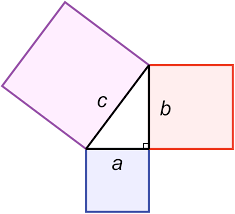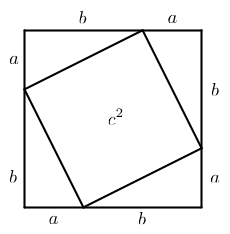# Pythagorean Theorem

The Pythagorean Theorem describes an important geometric relationship between the three sides of a right triangle. A right triangle is a triangle with a 90 degree angle (indicated by an 'L' shape). The longest side is called the hypotenuse which is across from the 90 degree angle. A right triangle has the following rule:

The square of the hypotenuse is equal to the sum of the squares of the other two sides.

By drawing a square on each side of the triangle, the equation says that the area of the largest square on the hypotenuse is equal to the sum of the area of the other squares.

The Pythagorean Theorem equation is:

$$\textcolor{blue}{a^2} + \textcolor{red}{b^2} = \textcolor{purple}{c^2}$$

where $$c$$ is the hypotenuse and $$a$$ and $$b$$ are the other sides called the opposite and adjacent sides (order does not matter).Integer values of $$a, b \; \text{and} \; c$$ that satisfy the equation are known as Pythagorean triples Some well known examples are (3,4,5) and (5,12,13). We can multiply the entries in a triple by any integer and get another triple such as (3,4,5) * 2 = (6,8,10), (3,4,5) * 3 = (9,12,15), (3,4,5) * 5 = (15,20,25) etc.

## Pythagorean Theorem Proof

The Pythagorean Theorem can also be proven simply with a diagram and a couple equations.

Refer to the diagram below. The big square with the side length of $$a + b$$ has encased a smaller square with length c. The remaining portions in the big square are triangles with lengths $$a$$ and $$b$$. Notice that these are right triangles!We can find the area of the big square as: $$A_{BigSquare} = (a + b) \cdot (a + b)$$

We can find the area of the small square as: $$A_{SmallSquare} = c^2$$

The area of each four triangles is: $$A_{Triangle} = \cfrac{a \cdot b}{2}$$

Notice that the area of the big square is equal to the area of smaller one plus 4 triangles: $$A_{BigSquare} = c^2 + \cfrac{a \cdot b}{2} \cdot 4$$

We can set the two equations for the big square equal to eachother:

$$(a + b) \cdot (a + b) = c^2 + 2 \cdot a \cdot b$$

Simplfying the equation gives:

$$a^2 + 2 \cdot a \cdot b + b^2 = c^2 + 2 \cdot a \cdot b$$

$$a^2 + b^2 = c^2$$

This is the Pythagorean Theorem!

We can use the Pythagorean Theorem to calculate the missing side of a triangle. Try a couple examples below!

What is the diagonal distance across a square of size 1?

In a right angle triangle, the hypotenuse is 15 m and opposite side is 12 m. What is the length of the triangle's adjacent side?

In a right angle triangle, the adjacent side is 20 km and opposite side is 15 km. What is the length of the triangle's hypotenuse?

A triangle with side length 26, 24 and 10. is this a right angle triangle?

A 15 foot ladder is put up against a building. The base of the ladder is 12 feet away from the building. How high will the ladder reach?